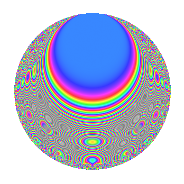# Properties

 Label 432.2.lLevel 432 Weight 2 Character orbit l Rep. character $$\chi_{432}(107,\cdot)$$ Character field $$\Q(\zeta_{4})$$ Dimension 64 Newform subspaces 2 Sturm bound 144 Trace bound 10

# Related objects

## Defining parameters

 Level: $$N$$ = $$432 = 2^{4} \cdot 3^{3}$$ Weight: $$k$$ = $$2$$ Character orbit: $$[\chi]$$ = 432.l (of order $$4$$ and degree $$2$$) Character conductor: $$\operatorname{cond}(\chi)$$ = $$48$$ Character field: $$\Q(i)$$ Newform subspaces: $$2$$ Sturm bound: $$144$$ Trace bound: $$10$$ Distinguishing $$T_p$$: $$5$$

## Dimensions

The following table gives the dimensions of various subspaces of $$M_{2}(432, [\chi])$$.

Total New Old
Modular forms 156 64 92
Cusp forms 132 64 68
Eisenstein series 24 0 24

## Trace form

 $$64q + O(q^{10})$$ $$64q + 4q^{10} - 28q^{16} - 8q^{19} + 20q^{22} + 12q^{28} - 12q^{34} - 36q^{40} + 16q^{43} + 16q^{46} + 64q^{49} - 12q^{52} + 32q^{55} - 48q^{58} + 16q^{61} + 24q^{64} - 64q^{67} - 72q^{70} - 144q^{76} - 40q^{82} + 16q^{85} + 12q^{88} - 24q^{91} - 36q^{94} + O(q^{100})$$

## Decomposition of $$S_{2}^{\mathrm{new}}(432, [\chi])$$ into newform subspaces

Label Dim. $$A$$ Field CM Traces $q$-expansion
$$a_2$$ $$a_3$$ $$a_5$$ $$a_7$$
432.2.l.a $$32$$ $$3.450$$ None $$0$$ $$0$$ $$0$$ $$0$$
432.2.l.b $$32$$ $$3.450$$ None $$0$$ $$0$$ $$0$$ $$0$$

## Decomposition of $$S_{2}^{\mathrm{old}}(432, [\chi])$$ into lower level spaces

$$S_{2}^{\mathrm{old}}(432, [\chi]) \cong$$ $$S_{2}^{\mathrm{new}}(48, [\chi])$$$$^{\oplus 3}$$$$\oplus$$$$S_{2}^{\mathrm{new}}(144, [\chi])$$$$^{\oplus 2}$$

## Hecke Characteristic Polynomials

There are no characteristic polynomials of Hecke operators in the database